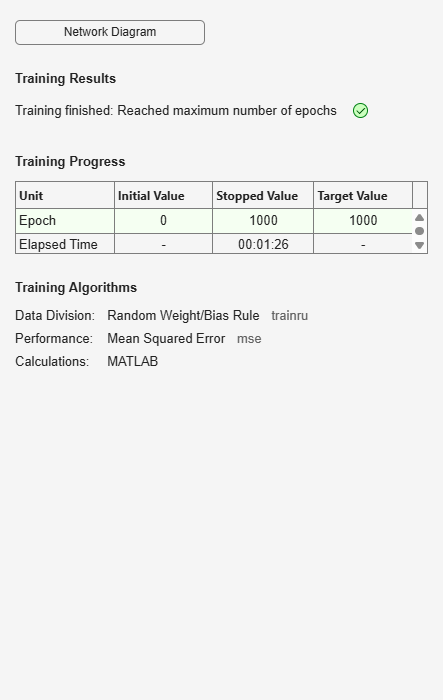# competlayer

Competitive layer

## Syntax

```competlayer(numClasses,kohonenLR,conscienceLR) ```

## Description

Competitive layers learn to classify input vectors into a given number of classes, according to similarity between vectors, with a preference for equal numbers of vectors per class.

`competlayer(numClasses,kohonenLR,conscienceLR)` takes these arguments,

 `numClasses` Number of classes to classify inputs (default = 5) `kohonenLR` Learning rate for Kohonen weights (default = 0.01) `conscienceLR` Learning rate for conscience bias (default = 0.001)

and returns a competitive layer with `numClasses` neurons.

## Examples

collapse all

This example shows how to train a competitive layer to classify 150 iris flowers into 6 classes.

```inputs = iris_dataset; net = competlayer(6); net = train(net,inputs);````view(net)````outputs = net(inputs); classes = vec2ind(outputs);```

## Version History

Introduced in R2010b